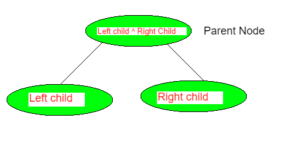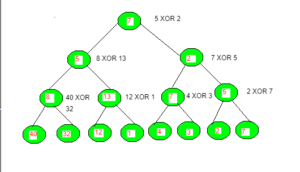GFG App
Open AppBrowser
Continue

# Construct XOR tree by Given leaf nodes of Perfect Binary Tree

Given the leaf nodes of a perfect binary tree, the task is to construct the XOR tree and print the root node of this tree.
An XOR tree is a tree whose parent node is the XOR of the left child and the right child node of the tree.
Parent node = Left child node ^ Right child nodeExamples:

Input: arr = {40, 32, 12, 1, 4, 3, 2, 7}
Output: Nodes of the XOR tree
7 5 2 8 13 7 5 40 32 12 1 4 3 2 7
Root: 7
Explanation:Input: arr = {5, 7, 2, 8, 12, 3, 9, 1}
Output: Nodes of the XOR tree
15 8 7 2 10 15 8 5 7 2 8 12 3 9 1
Root: 15

Input: arr = {4, 2, 10, 1, 14, 30, 21, 7}
Output: Nodes of the XOR tree
15 13 2 6 11 16 18 4 2 10 1 14 30 21 7
Root: 15

Input: arr = {1, 2, 3, 4}
Output: Nodes of the XOR tree
4 3 7 1 2 3 4
Root: 4

Input: arr = {47, 62, 8, 10, 4, 3, 1, 7}
Output: Nodes of the XOR tree
18 19 1 17 2 7 6 47 62 8 10 4 3 1 7
Root: 18

Approach:

1. Since it’s a perfect binary tree, there are a total of 2^h-1 nodes, where h is the height of the XOR tree.
2. Since leaf nodes of a perfect binary tree are given, the root node is built by first building the left and right subtrees recursively.
3. Every node in the left and right subtrees is formed by performing the XOR operation on their children.

Below is the implementation of the above approach.

## C++

 `// C++ implementation of the above approach` `#include ` `using` `namespace` `std;`   `// Maximum size for xor tree` `int` `maxsize = 100005;`   `// Allocating space to xor tree` `vector<``int``> xor_tree(maxsize);`   `// A recursive function that constructs xor tree` `// for vector array[start.....end].` `// x is index of current node in XOR tree`   `void` `construct_Xor_Tree_Util(vector<``int``> current,` `                             ``int` `start, ``int` `end, ``int` `x)` `{` `    ``// If there is one element in vector array, store it` `    ``// in current node of XOR tree` `    ``if` `(start == end) {` `        ``xor_tree[x] = current[start];` `        ``// cout< arr, ``int` `n)` `{` `    ``construct_Xor_Tree_Util(arr, 0, n - 1, 0);` `}`   `// Driver Code` `int` `main()` `{`   `    ``// leaf nodes  of Perfect Binary Tree` `    ``vector<``int``> leaf_nodes = { 40, 32, 12, 1, 4, 3, 2, 7 };`   `    ``int` `n = leaf_nodes.size();`   `    ``// Build the xor tree` `    ``construct_Xor_Tree(leaf_nodes, n);`   `    ``// Height of xor tree` `    ``int` `x = (``int``)(``ceil``(log2(n)));`   `    ``// Maximum size of xor tree` `    ``int` `max_size = 2 * (``int``)``pow``(2, x) - 1;`   `    ``cout << ``"Nodes of the XOR Tree:\n"``;` `    ``for` `(``int` `i = 0; i < max_size; i++) {` `        ``cout << xor_tree[i] << ``" "``;` `    ``}`   `    ``// Root node is at index 0 considering` `    ``// 0-based indexing in XOR Tree` `    ``int` `root = 0;`   `    ``// print value at root node` `    ``cout << ``"\nRoot: "` `<< xor_tree[root];` `}`

## C

 `// C program to build xor tree by leaf nodes` `// of perfect binary tree and root node value of tree`   `#include ` `#include `   `// maximum size for xor tree` `#define maxsize 10005`   `// Allocating space to xor tree` `int` `xortree[maxsize];`   `// A recursive function that constructs xor tree` `// for  array[start.....end].` `// x is index of current node in xor tree st` `void` `construct_Xor_Tree_Util(``int` `current[],` `                             ``int` `start, ``int` `end, ``int` `x)` `{` `    ``// If there is one element in array, store it` `    ``// in current node of xor tree and return` `    ``if` `(start == end) {` `        ``xortree[x] = current[start];` `        ``// printf("%d ", xortree[x]);` `        ``return``;` `    ``}` `    ``// for left subtree` `    ``int` `left = x * 2 + 1;` `    ``// for right subtree` `    ``int` `right = x * 2 + 2;`   `    ``// for getting the middle index` `    ``// from corner indexes.` `    ``int` `mid = start + (end - start) / 2;`   `    ``// Build the left and the right subtrees` `    ``// by xor operation` `    ``construct_Xor_Tree_Util(current, start, mid, left);`   `    ``construct_Xor_Tree_Util(current, mid + 1, end, right);`   `    ``// merge the left and right subtrees by` `    ``// XOR operation`   `    ``xortree[x] = (xortree[left] ^ xortree[right]);` `}`   `// Function to construct XOR tree from given array.` `// This function calls construct_Xor_Tree_Util()` `// to fill the allocated memory of xort  array` `void` `construct_Xor_Tree(``int` `arr[], ``int` `n)` `{` `    ``int` `i = 0;` `    ``for` `(i = 0; i < maxsize; i++)` `        ``xortree[i] = 0;` `    ``construct_Xor_Tree_Util(arr, 0, n - 1, 0);` `}`   `// Driver Code` `int` `main()` `{`   `    ``// leaf nodes of Binary Tree` `    ``int` `leaf_nodes[] = { 40, 32, 12, 1, 4, 3, 2, 7 }, i = 0;`   `    ``int` `n = ``sizeof``(leaf_nodes) / ``sizeof``(leaf_nodes);`   `    ``// Build the xor tree` `    ``construct_Xor_Tree(leaf_nodes, n);`   `    ``// Height of xor tree` `    ``int` `x = (``int``)(``ceil``(log2(n)));`   `    ``// Maximum size of xor tree` `    ``int` `max_size = 2 * (``int``)``pow``(2, x) - 1;`   `    ``printf``(``"Nodes of the XOR tree\n"``);` `    ``for` `(i = 0; i < max_size; i++) {` `        ``printf``(``"%d "``, xortree[i]);` `    ``}`   `    ``// Root node is at index 0 considering` `    ``// 0-based indexing in XOR Tree` `    ``int` `root = 0;`   `    ``// print value at root node` `    ``printf``(``"\nRoot: %d"``, xortree[root]);` `}`

## Java

 `// Java implementation of the above approach` `class` `GFG` `{`   `// Maximum size for xor tree` `static` `int` `maxsize = ``100005``;`   `// Allocating space to xor tree` `static` `int` `[]xor_tree = ``new` `int``[maxsize];`   `// A recursive function that constructs xor tree` `// for vector array[start.....end].` `// x is index of current node in XOR tree`   `static` `void` `construct_Xor_Tree_Util(``int` `[]current,` `                            ``int` `start, ``int` `end, ``int` `x)` `{` `    ``// If there is one element in vector array, store it` `    ``// in current node of XOR tree` `    ``if` `(start == end)` `    ``{` `        ``xor_tree[x] = current[start];` `        `  `        ``// System.out.print(xor_tree[x]+" x";` `        ``return``;` `    ``}` `    `  `    ``// for left subtree` `    ``int` `left = x * ``2` `+ ``1``;` `    `  `    ``// for right subtree` `    ``int` `right = x * ``2` `+ ``2``;`   `    ``// for getting the middle index from corner indexes.` `    ``int` `mid = start + (end - start) / ``2``;`   `    ``// Build the left and the right subtrees by xor operation` `    ``construct_Xor_Tree_Util(current, start, mid, left);`   `    ``construct_Xor_Tree_Util(current, mid + ``1``, end, right);`   `    ``// merge the left and right subtrees by` `    ``// XOR operation`   `    ``xor_tree[x] = (xor_tree[left] ^ xor_tree[right]);` `}`   `// Function to conXOR tree from the given vector array.` `// This function calls construct_Xor_Tree_Util() to fill the` `// allocated memory of xor_tree vector array` `static` `void` `construct_Xor_Tree(``int` `[]arr, ``int` `n)` `{` `    ``construct_Xor_Tree_Util(arr, ``0``, n - ``1``, ``0``);` `}`   `// Driver Code` `public` `static` `void` `main(String[] args)` `{`   `    ``// leaf nodes of Perfect Binary Tree` `    ``int` `[]leaf_nodes = { ``40``, ``32``, ``12``, ``1``, ``4``, ``3``, ``2``, ``7` `};`   `    ``int` `n = leaf_nodes.length;`   `    ``// Build the xor tree` `    ``construct_Xor_Tree(leaf_nodes, n);`   `    ``// Height of xor tree` `    ``int` `x = (``int``)(Math.ceil(Math.log(n)));`   `    ``// Maximum size of xor tree` `    ``int` `max_size = ``2` `* (``int``)Math.pow(``2``, x) - ``1``;`   `    ``System.out.print(``"Nodes of the XOR Tree:\n"``);` `    ``for` `(``int` `i = ``0``; i < max_size; i++)` `    ``{` `        ``System.out.print(xor_tree[i]+ ``" "``);` `    ``}`   `    ``// Root node is at index 0 considering` `    ``// 0-based indexing in XOR Tree` `    ``int` `root = ``0``;`   `    ``// print value at root node` `    ``System.out.print(``"\nRoot: "` `+ xor_tree[root]);` `}` `}`   `// This code is contributed by PrinciRaj1992`

## Python

 `# Python3 implementation of the above approach` `from` `math ``import` `ceil,log`   `# Maximum size for xor tree` `maxsize ``=` `100005`   `# Allocating space to xor tree` `xor_tree ``=` `[``0``] ``*` `maxsize`   `# A recursive function that constructs xor tree` `# for vector array[start.....end].` `# x is index of current node in XOR tree` `def` `construct_Xor_Tree_Util(current, start, end, x):` `    `  `    ``# If there is one element in vector array, store it` `    ``# in current node of XOR tree` `    ``if` `(start ``=``=` `end):` `        ``xor_tree[x] ``=` `current[start]` `        `  `        ``# cout<

## C#

 `// C# implementation of the above approach` `using` `System;` `using` `System.Collections.Generic;`   `class` `GFG` `{`   `// Maximum size for xor tree` `static` `int` `maxsize = 100005;`   `// Allocating space to xor tree` `static` `int` `[]xor_tree = ``new` `int``[maxsize];`   `// A recursive function that constructs xor tree` `// for vector array[start.....end].` `// x is index of current node in XOR tree` `static` `void` `construct_Xor_Tree_Util(``int` `[]current,` `                            ``int` `start, ``int` `end, ``int` `x)` `{` `    ``// If there is one element in vector array, store it` `    ``// in current node of XOR tree` `    ``if` `(start == end)` `    ``{` `        ``xor_tree[x] = current[start];` `        `  `        ``// Console.Write(xor_tree[x]+" x";` `        ``return``;` `    ``}` `    `  `    ``// for left subtree` `    ``int` `left = x * 2 + 1;` `    `  `    ``// for right subtree` `    ``int` `right = x * 2 + 2;`   `    ``// for getting the middle index from corner indexes.` `    ``int` `mid = start + (end - start) / 2;`   `    ``// Build the left and the right subtrees by xor operation` `    ``construct_Xor_Tree_Util(current, start, mid, left);`   `    ``construct_Xor_Tree_Util(current, mid + 1, end, right);`   `    ``// merge the left and right subtrees by` `    ``// XOR operation` `    ``xor_tree[x] = (xor_tree[left] ^ xor_tree[right]);` `}`   `// Function to conXOR tree from the given vector array.` `// This function calls construct_Xor_Tree_Util() to fill the` `// allocated memory of xor_tree vector array` `static` `void` `construct_Xor_Tree(``int` `[]arr, ``int` `n)` `{` `    ``construct_Xor_Tree_Util(arr, 0, n - 1, 0);` `}`   `// Driver Code` `public` `static` `void` `Main(String[] args)` `{`   `    ``// leaf nodes of Perfect Binary Tree` `    ``int` `[]leaf_nodes = { 40, 32, 12, 1, 4, 3, 2, 7 };`   `    ``int` `n = leaf_nodes.Length;`   `    ``// Build the xor tree` `    ``construct_Xor_Tree(leaf_nodes, n);`   `    ``// Height of xor tree` `    ``int` `x = (``int``)(Math.Ceiling(Math.Log(n)));`   `    ``// Maximum size of xor tree` `    ``int` `max_size = 2 * (``int``)Math.Pow(2, x) - 1;`   `    ``Console.Write(``"Nodes of the XOR Tree:\n"``);` `    ``for` `(``int` `i = 0; i < max_size; i++)` `    ``{` `        ``Console.Write(xor_tree[i] + ``" "``);` `    ``}`   `    ``// Root node is at index 0 considering` `    ``// 0-based indexing in XOR Tree` `    ``int` `root = 0;`   `    ``// print value at root node` `    ``Console.Write(``"\nRoot: "` `+ xor_tree[root]);` `}` `}`   `// This code is contributed by PrinciRaj1992`

## Javascript

 ``

Output:

```Nodes of the XOR Tree:
7 5 2 8 13 7 5 40 32 12 1 4 3 2 7
Root: 7```

Time Complexity: O(N)
We need to traverse all the leaf nodes in the XOR tree to construct it. So the time complexity is O(N) where N is the number of leaf nodes.

Space Complexity: O(N)
We need to create a vector of size N to store the XOR tree. Hence, the space complexity is O(N).

My Personal Notes arrow_drop_up